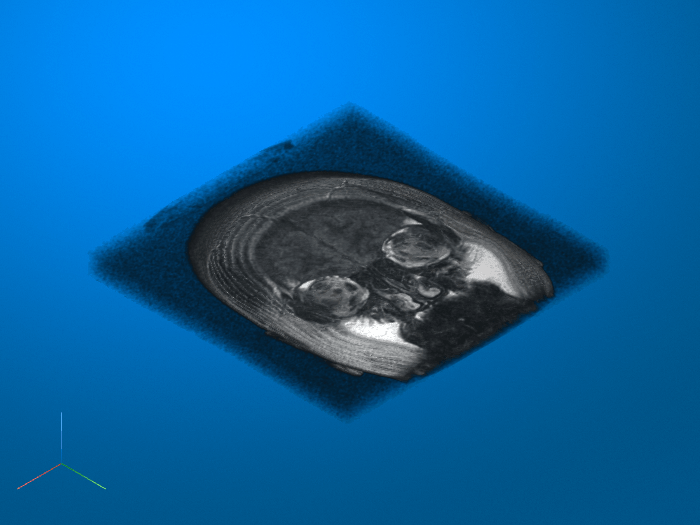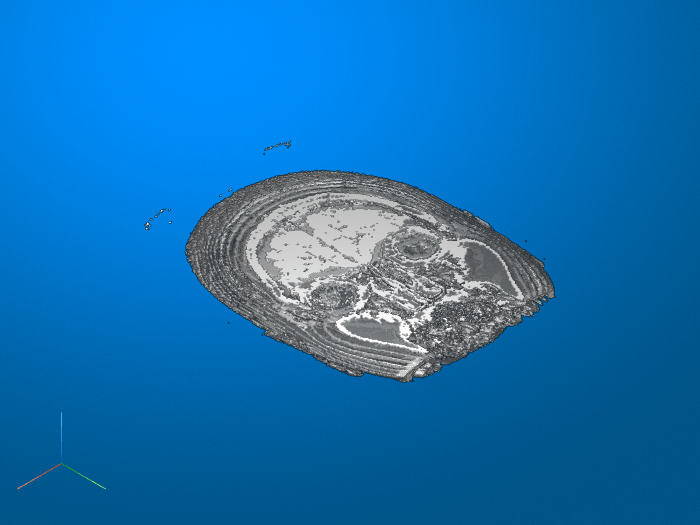# imsegkmeans3

K-means clustering based volume segmentation

## Syntax

``L = imsegkmeans3(V,k)``
``[L,centers] = imsegkmeans3(V,k)``
``L = imsegkmeans3(V,k,Name,Value)``

## Description

example

````L = imsegkmeans3(V,k)` segments volume `V` into `k` clusters by performing k-means clustering and returns the segmented labeled output in `L`.```
````[L,centers] = imsegkmeans3(V,k)` also returns the cluster centroid locations, `centers`.```
````L = imsegkmeans3(V,k,Name,Value)` uses name-value pairs to control aspects of the k-means clustering algorithm.```

## Examples

collapse all

Load a 3-D grayscale MRI volume and display it using `volshow`.

```load mristack volshow(mristack);```Segment the volume into three clusters.

`L = imsegkmeans3(mristack,3);`

Display the segmented volume using `volshow`. To explore slices of the segmented volume, use the Volume Viewer app.

```figure volshow(L);```## Input Arguments

collapse all

Volume to segment, specified as a 3-D grayscale volume of size m-by-n-by-p or a 3-D multispectral volume of size m-by-n-by-p-by-c, where p is the number of planes and c is number of channels.

Note

`imsegkmeans3` treats 2-D color images like 3-D volumes of size m-by-n-by-3. If you want 2-D behavior, then use the `imsegkmeans` function.

Data Types: `single` | `int8` | `int16` | `uint8` | `uint16`

Number of clusters to create, specified as a positive integer.

### Name-Value Arguments

Specify optional pairs of arguments as `Name1=Value1,...,NameN=ValueN`, where `Name` is the argument name and `Value` is the corresponding value. Name-value arguments must appear after other arguments, but the order of the pairs does not matter.

Before R2021a, use commas to separate each name and value, and enclose `Name` in quotes.

Example: `'NumAttempts',5`

Normalize input data to zero mean and unit variance, specified as the comma-separated pair consisting of `'NormalizeInput'` and a numeric or logical `1` (`true`) or `0` (`false`). If you specify `true`, then `imsegkmeans3` normalizes each channel of the input individually.

Number of times to repeat the clustering process using new initial cluster centroid positions, specified as the comma-separated pair consisting of `'NumAttempts'` and a positive integer.

Maximum number of iterations, specified as the comma-separated pair consisting of `'MaxIterations'` and a positive integer.

Accuracy threshold, specified as the comma-separated pair consisting of `'Threshold'` and a positive number. The algorithm stops when each of the cluster centers move less than the threshold value in consecutive iterations.

## Output Arguments

collapse all

Label matrix, specified as a matrix of positive integers. Pixels with label 1 belong to the first cluster, label 2 belong to the second cluster, and so on for each of the `k` clusters. `L` has the same first three dimensions as volume `V`. The class of `L` depends on number of clusters.

Class of `L`Number of Clusters
`'uint8'``k <= 255`
`'uint16'``256 <= k <= 65535`
`'uint32'``65536 <= k <= 2^32-1`
`'double'``2^32 <= k`

Cluster centroid locations, returned as a numeric matrix of size k-by-c, where k is the number of clusters and c is the number of channels. `centers` is the same class as the image `I`.

## Tips

• The function yields reproducible results. The output will not vary in multiple runs given the same input arguments.

 Arthur, David, and Sergei Vassilvitskii. “K-Means++: The Advantages of Careful Seeding.” In Proceedings of the Eighteenth Annual ACM-SIAM Symposium on Discrete Algorithms, 1027–35. SODA ’07. USA: Society for Industrial and Applied Mathematics, 2007.